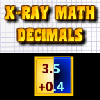Custom Search

# Find Our Cool Math Games to Play by Category:

<<<<< PREVIOUS 6.NS.B.3 GAMES

### 6.NS.B.3

Fluently add, subtract, multiply, and divide multi-digit decimals using the standard algorithm for each operation.CCSS: 5.NBT.B.7, 6.NS.B.3
Make your panda run faster and beat the other running pandas by answering the decimal problems correctly.  Put your decimal skills to the test and complete all of the challenging levels.CCSS: 4.NF.A.1, 5.NBT.B.7, 6.NS.B.3, 7.NS.A.1d, 7.NS.A.2c, 7.NS.A.2d
Snake Math Advanced Basic too easy for you?  Then play this game, Snake Math Advanced, for a real challenge!  Just like Snake Math Advanced Basic but a little harder.CCSS: 4.NF.A.1, 5.NBT.B.7, 6.NS.B.3, 7.NS.A.1d, 7.NS.A.2c, 7.NS.A.2d
Like Snake Math above but in this game you get to choose to play using equivalent fractions, fractions to decimals, decimal operations or positive/negative integer operations.CCSS: 5.NBT.B.7, 6.NS.B.3
The version of X-ray math containing decimal problems.  Choose a box and slide it under the X-ray bar to reveal the decimal problem.  Then move the box to its answer.  Take your time as there are no time limits in this game.

<<<<< PREVIOUS 6.NS.B.3 GAMES